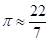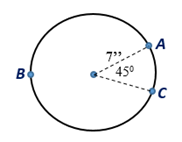Chapter 8.CT, Problem 11CT### Elementary Geometry for College St...

6th Edition
Daniel C. Alexander + 1 other
ISBN: 9781285195698

#### Solutions

Chapter
Section### Elementary Geometry for College St...

6th Edition
Daniel C. Alexander + 1 other
ISBN: 9781285195698
Textbook Problem
1 views

# Where, find the approximate length of A C ^ . l A C ^ ≈                                   _To determine

To find:

The approximate length of AC^.

Explanation

Given:

If π227 and the figure given below.

Theorem:

1. The circumference C of a circle is given by the formula, C=πd or C=2πr where d is the diameter and r is the radius of the circle.

2. In a circle whose circumference is C, the length l of an arc whose degree measure is m is given by l=m360·C.

From the given, π227.

Form the figure, m=45, and r=7 in.

To find the circumference of the circle, substitute r=7 in C=2πr

### Still sussing out bartleby?

Check out a sample textbook solution.

See a sample solution

#### The Solution to Your Study Problems

Bartleby provides explanations to thousands of textbook problems written by our experts, many with advanced degrees!

Get Started

#### Find the maximum revenue R (in dollars) given that 12(2R 320) 4(3R + 240)

Applied Calculus for the Managerial, Life, and Social Sciences: A Brief Approach

#### Find f'(x) and f"(x). f(x) = (x3 + 1)ex

Single Variable Calculus: Early Transcendentals

#### In Problems 1-8, find the derivative of each function.

Mathematical Applications for the Management, Life, and Social Sciences

#### True or False: The function graphed at the right is one-to-one.

Study Guide for Stewart's Single Variable Calculus: Early Transcendentals, 8th